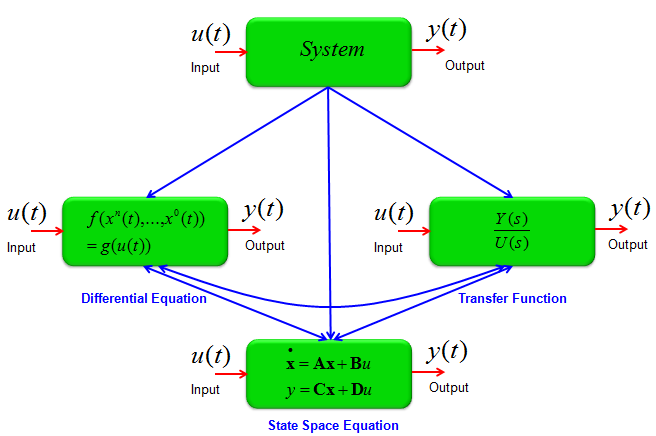DE - Modeling                                                                          Home : www.sharetechnote.com What is Modeling ?   Modeling in differential equation refers to a process of finding mathematical equation (differential equation) that explains/describes a specific situation.   Most of the mathematical methods are designed to express a real life problems into a mathematical language. This is called 'modeling', at least in engineering Mathematical Modeling is the most important reason why we have to study math.   In most of classroom in school, most of the focus is placed on how to solve a given differential problem. But as I mentioned in other posts, I think it is more important to understand the meaning of the given equation and it would be the best if you can derive the equation on your own from a given situation.     What is the most important steps for Modeling ?   The most important thing for modeling is to figure out the governing equation for the given situation (problem). The governing equation varies depending the area/field, so you would need to have the basic understandings on theory for the given problem. You may get the basic idea from Governing Equation page, but you would learn the governing equation (law) for each specific cases in all of the modeling examples.     What kind of mathematical form for a model ?   Basically you can represent a given problem into any form of mathematical model and it is completely up to you to pick up which form you want to use, but following illustration would show you some of commonly used mathematical model.   With Matrix, Differential Equation is one of the most usefull tool for mathematical modeling and following illustration would show you a several different format of Mathematical Models derived from differential equation.Usually (at least, in most of University Courses), the first step is to describe a system into a set of differential equations and convert those equations into Transfer Function (by Laplace Transform) and State Space Equations. So it is very important to know the concept of differential equations, but as you repeat this kind of modeling process or work in a specific field for a long time, you would get the tricks to directly construct Transfer Function or State Space Equation without getting into the steps of building up the differential equation.   There are many engineering softwares out there to solve various engineering problems. Some of the tools requires you to input your problem in the form of differential equation, some others requires the input as Transfer Function and some others requires the input as State Space Equation. To utilize these tools as much as possible, you need to understand the concept of all these mathematical variations.